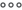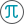You can add equations to a file. Equations are entered in the LaTeX markup language and are automatically converted and displayed in the text in a standard (conventional) form.

To insert an equation, do the following:

1.Place the cursor where you want to insert an equation.

2.Execute the Insert command in one of the following ways:

In the Command menu, select Insert > Equation.

On the Toolbar, select the Insert section and click. On the displayed insert pane, clickEquation.

3.In the Equation window, in the LaTeX Expression box, enter the equation in the LaTeX markup language. You can preview the equation in the Preview box.

4.Click ОК.

In the current version of the application it is impossible to display the equations created in Microsoft Word 2003 and 2007.
Was the material useful?
Yes
No